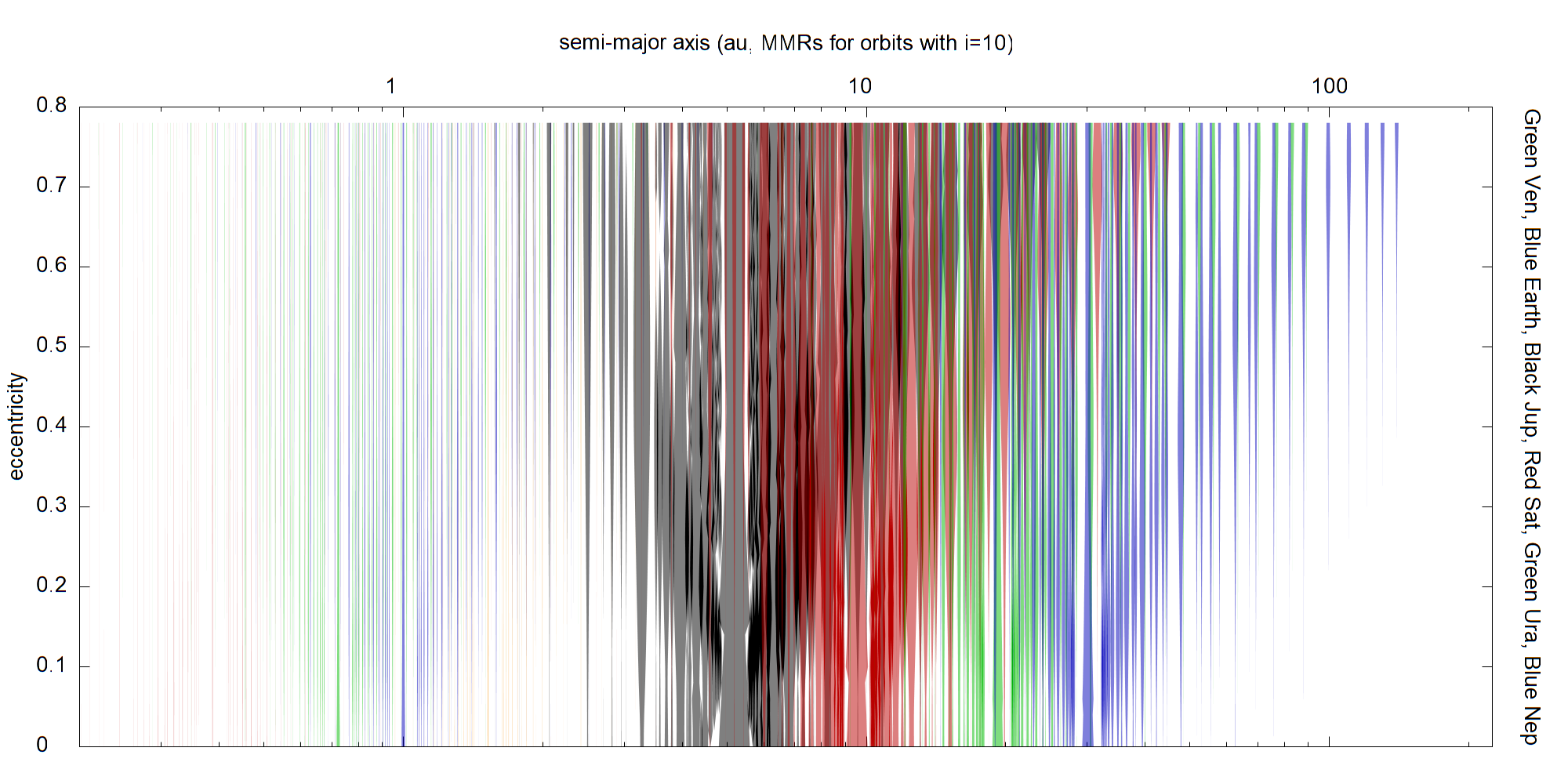## ATLAS OF TWO BODY MEAN MOTION RESONANCES IN THE SOLAR SYSTEM VERSION 2020

Tabare Gallardo
Departamento Astronomia, Facultad de Ciencias, Uruguay
www.fisica.edu.uy/~gallardo
gallardo(at)fisica.edu.uy### Summary

• The present plots, tables and programs give the locations in au, strengths, widths (of stable librations) in au, libration periods in years and libration centers in degrees of the MMRs kp:k (kp*np = k*n) between a massless particle and the planets.
• No restrictions in eccentricity nor in inclination: you can calculate MMRs of polar, retrograde and sungrazer objects, for example.
• Widths, libration periods and centers are not universal and depend on the orbital elements (mainly e,i,w) of the asteroid/comet/centaur/TNO (or test particle).
• MMRs are calculated considering fixed orbits for both particle and planet.
• Mean semimajor axes and mean eccentricities were considered for the planets which were assumed with i=0 and varpi_p=0 (varpi is the longitude of the perihelion).
• Calculations are based on the article by Gallardo 2020, (arXiv) for planets in circular orbits and generalized to planets in eccentric orbits.
• EXOPLANETARY SYSTEMS: the codes are general, valid for our system and also for extrasolar systems, just put the right data in the input file plasys.inp

### Plots

Plots in the plane (a,e) showing the domains (widths of the stable librations) of the MMRs for test particles with i=10. Superposition of resonances means chaos.
Plot (a,width) for test particles with e=0.15, i=8 (typical of asteroids). Height of the lines represent the width in au. Code colour indicates the planet responsible for the resonance: Mercury=red, Venus=green, Earth=blue, Mars=yellow, Jupiter=black, Saturn=red, Uranus=green, Neptune=blue.
Some examples showing the inclination effect. All resonances kp:k with kp <= 10, k <= 10.

### Tables

All resonances verifying kp <= 20, k <= 20 with all the planets, calculated for typical (e,i) values of asteroids (e=0.15, i=8)

### Programs

• Resonalyzerv2. To compute the resonant disturbing function R(sigma) and to calculate the location in semimajor axis, its strength SR, maximum stable width in au, stable equilibrium points and corresponding libration periods in years. The code takes into account the eccentricity of the planet if you want (that is why the code is v2). IMPORTANT: in the input file plasys.inp you must state the data of the central star and planetary system.
• Superatlasv2. Calculates an atlas of resonances in some interval of semimajor axis considering planetary eccentricities. IMPORTANT: in the input file plasys.inp you must state the data of the central star and planetary system.

### Usage

Suppose you are studying the dynamics of a small body and you want to know if it is captured in a resonant motion. You have two possibilities:
• Look at the table for the strongest resonances near the semimayor axis of the body's orbit. Then, using your numerical integration, compute the corresponding critical angles
sigma = k*lambda - kp*lambda_planet + (kp-k)*varpi
(varpi=argument of the perihelion + longitude of the node) and follow the time evolution.
• Or run the program Superatlasv2 and compute the strength according to the (e,i,w,ln) where ln is in fact the difference long of the node asteroid - long of the perihelion planet, (OK, you can put arbitrary value for ln) This is the best choice. Then compute the time evolution of the critical angle(s).

### Conventions

```   resonance kp:k verify kp*np = k*n

if kp > k is an interior resonance (3:2 the Hildas)
if kp < k is an exterior resonance (2:3 the plutinos)

principal critical angle:

sigma = k*lambda - kp*lambda_planet + (kp-k)*varpi
lambda = longper + mean anomaly
varpi = longper = longnod + argper
```

### Limitations

The general description of the MMRs obtained with the codes are quite good. Only one planet is taken into account for each calculation then secular or resonant effects due to other planets can modify the results. The metod assumes that the borders of the resonance are symmetric with respect to the nominal position of the resonance.